### 44道JavaScript送命题

1个月前 (04-26) 投稿作者：小石头资源网 围观人数：111 评论人数：0次# 1. map&parseInt传参

["1", "2", "3"].map(parseInt)结果是什么？

map方法指定一个回调函数，重新创建一个由回调函数返回值组成的新数组。该方法的原型是：

```var new_array = arr.map(function callback(currentValue[, index[, array]]) {
// Return element for new_array
}[, thisArg])```

map接受2个参数，一个是回调函数callback，一个是回调函数的this值。

• callback：生成新数组元素的函数，有三个参数
• currentValue：callbac当前正在处理的元素
• index：callback当前正在处理的当前元素的索引
• array：map方法调用的数组本身
• thisArg：执行callback函数时值被当做this

parseInt('1', 0);
parseInt('2', 1);
parseInt('3', 2);

# 2. typeof和instanceof

null是所有原型链的最顶端，null instanceof Object返回false，假设null这个值有构造函数Null，obj instanceof Null才会返回true。

# 3.reduce&Math.pow传参

和第1题有些类似。arr.reduce方法对数组中每个元素执行一个自定义的reducer函数（升序执行），并将结果汇总为单个值，这个常常用来累加一个数组中的数字。原型如下：

`arr.reduce(callback(accumulator, currentValue[, index[, array]])[, initialValue]) `
• callback：数组中每个值要执行的函数，有四个参数。
• accumulator：它是上一次回调函数时得到的值，或者initialValue。
• currentValue：数组中正在处理的当前元素。
• index：可选，数组中正在处理的元素的索引，如果提供了initialValue，则起始索引号为0，否则从索引1开始。
• array：调用reduce()的数组
• initialValue：作为第一次调用callback时的第一个参数的值。如果没有提供初始值，则将使用数组中的第一个元素。在没有初始值的空数组上调用reduce将报错。

1. 如果调用`reduce()`时提供了`initialValue``accumulator`取值为`initialValue``currentValue`取数组中的第一个值；如果没有提供 `initialValue`，那么`accumulator`取数组中的第一个值，`currentValue`取数组中的第二个值。
2. 如果数组为空且没有提供initialValue，会抛出TypeError 。如果数组仅有一个元素（无论位置如何）并且没有提供initialValue， 或者有提供initialValue但是数组为空，那么此唯一值将被返回并且callback不会被执行。

Math.pow(base, exponent)，返回基数base的指数exponent次幂，即baseexponent。

# 4. 优先级

```var val = 'smtg';
console.log('Value is ' + (val === 'smtg') ? 'Something' : 'Nothing'); ```

`console.log('Value is true' ? 'Something' : 'Nothing'); `

# 5.变量提升问题

```var name = 'World!';
(function () {
if (typeof name === 'undefined') {
var name = 'Jack';
console.log('Goodbye ' + name);
} else {
console.log('Hello ' + name);
}
})(); ```

```var name = 'World!';
(function () {
var name = undefined;
if (typeof name === 'undefined') {
var name = 'Jack';
console.log('Goodbye ' + name);
} else {
console.log('Hello ' + name);
}
})(); ```

name的声明放在了函数顶部，但是值是undefined。因为代码又放在一个闭包里，用外层那个name = “world”是不能访问的，闭包有隔离变量的作用。最后，上面的语句输出“Goodbye Jack”。

# 6. JavaScript能表示的最大数

```var END = Math.pow(2, 53);
var START = END - 100;
var count = 0;
for (var i = START; i <= END; i++) {
count++;
}
console.log(count); ```

```    var END = Math.pow(2, 53);
var START = END - 5;
var count = 0;
console.log(END);                   //      9007199254740992
console.log(END + 7);               //      9007199254740997
console.log(START++ <= END, START); // true 9007199254740988
console.log(START++ <= END, START); // true 9007199254740989
console.log(START++ <= END, START); // true 9007199254740990
console.log(START++ <= END, START); // true 9007199254740991
console.log(START++ <= END, START); // true 9007199254740992
console.log(START++ <= END, START); // true 9007199254740992
console.log(START++ <= END, START); // true 9007199254740992
console.log(START++ <= END, START); // true 9007199254740992
console.log(START++ <= END, START); // true 9007199254740992
console.log(START++ <= END, START); // true 9007199254740992
console.log(START++ <= END, START); // true 9007199254740992
console.log(START++ <= END, START); // true 9007199254740992 ```

# 7. 稀疏数组问题

```var ary = [0,1,2];
ary = 10;
var result = ary.filter(function(x) { return x === undefined;});```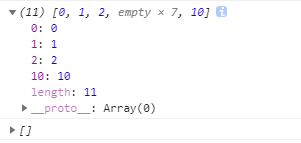# 8. 数字精度问题

```var two   = 0.2;
var one   = 0.1;
var eight = 0.8;
var six   = 0.6;
console.log([two - one === one, eight - six === two])```

# 9. 字面量问题

```function showCase(value) {
switch(value) {
case 'A':
console.log('Case A');
break;
case 'B':
console.log('Case B');
break;
case undefined:
console.log('undefined');
break;
default:
console.log('Do not know!');
}
}
showCase(new String('A'));```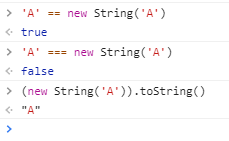# 10. String()函数

```function showCase2(value) {
switch(value) {
case 'A':
console.log('Case A');
break;
case 'B':
console.log('Case B');
break;
case undefined:
console.log('undefined');
break;
default:
console.log('Do not know!');
}
}
showCase2(String('A')); ```

# 11. 除法运算符%

```function isOdd(num) {
return num % 2 == 1;
}
function isEven(num) {
return num % 2 == 0;
}
function isSane(num) {
return isEven(num) || isOdd(num);
}
var values = [7, 4, '13', -9, Infinity];
values.map(isSane); ```

# 12. parseInt

```console.log(parseInt(3, 8));
console.log(parseInt(3, 2));
console.log(parseInt(3, 0)); ```

# 13. Array.prototype

`console.log(Array.isArray(Array.prototype)); `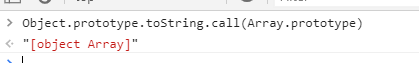所以本题的答案是true。

# 14. if语句

```var a = ;
if () {
console.log(a == true);
} else {
console.log("wut");
} ```

# 15. 对象比较问题

`[]==[] `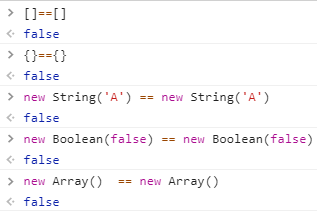上面比较对象（复杂数据）得到的结果都是false，所以本题结果是false。

# 16. +是字符串连接符也是加法运算

```console.log('5' + 3);
console.log('5' - 3); ```

# 17. 加减运算和正负运算

`console.log(1 + - + + + - + 1); `

# 18. 还是稀疏数组

```var ary = Array(3);
ary=2
let s = ary.map(function(elem) { return '1'; });
console.log(s); ```

# 19. argument对象

```function sidEffecting(ary) {
arguments = 10;
ary = ary;
}
function bar(a,b,c) {
c = 10
sidEffecting(arguments);
return a + b + c;
}
console.log(bar(1,1,1)) ```

# 20. JavaScript中的最大数

```var a = 111111111111111110000,
b = 1111;
console.log(a + b); ```

# 21. Array.property.reverse

```var x = [].reverse;
x(); ```

# 22. Number.MIN_VALUE

`console.log(Number.MIN_VALUE > 0); `

Number.MIN_VALUE表示最小正数，即最接近 0 的正数 (实际上不会变成 0)。最大的负数是 `-MIN_VALUE`

# 23. 强制转换

`console.log([1 < 2 < 3, 3 < 2 < 1]); `

# 24. 数组字面量的字符串表示

`console.log(2 == [[]]); `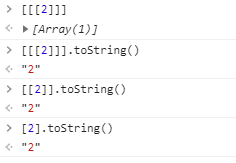# 25. 3.和.3

```console.log(3.toString());
console.log(3..toString());
console.log(3...toString()); ```

# 26. var和闭包问题

```(function(){
var x = y = 1;
})();
console.log(y);
console.log(x); ```

# 27. 正则表达式不可相互比较

```var a = /123/, b = /123/;
console.log(a == b);
console.log(a === b); ```

# 28. 数组比较

```    var a = [1, 2, 3], b = [1, 2, 3], c = [1, 2, 4];
console.log(a ==  b);
console.log(a === b);
console.log(a > c);
console.log(a < c); ```

• 比较a<c，相等，继续比较下一位
• 比较a<c，相等，继续比较下一位
• 比较a<c，返回true，于是a<c返回true

# 29. 构造函数的原型

```var a = {}, b = Object.prototype
console.log([a.prototype === b, Object.getPrototypeOf(a) === b]); ```

JavaScript中函数才有prototype属性，对象是没有的，对象有__proto__，指向对象的构造函数的原型，所以a.prototype是undefined。b是Object函数的原型，是一个对象，所以第一个是false。第二个是使用getPrototypeOf方法获取对象a的原型（即a.__proto__），这个和Object.prototype相等的，所以第二个表达式返回true。

a.__proto__是对象的原型，它是浏览器暴露出来的属性，JavaScript标准中没有这个属性。下面的语句输出[true]：

```var a = {}, b = Object.prototype
console.log([a.__proto__ === b); ```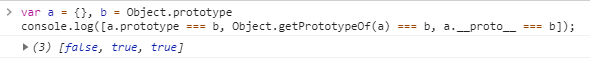# 30. 函数的原型对象

```function f() {}
var a = f.prototype, b = Object.getPrototypeOf(f);
console.log(a === b); ```

# 31. Function.name

```function foo() { }
var oldName = foo.name;
foo.name = "bar";
console.log([oldName, foo.name]); ```

# 32. str.replace

`console.log("1 2 3".replace(/\d/g, parseInt)); `

`str.replace(regexp|substr, newSubStr|function) `

• regexp (pattern)：一个正则表达式对象（即RegExp对象）或者其字面量。该正则所匹配的内容会被第二个参数的返回值替换掉。它和substr是二选一的。
• substr (pattern)：一个将被newSubStr替换的字符串。其被视为一整个字符串，而不是一个正则表达式。仅第一个匹配项会被替换。它和regexp是二选一的。
• newSubStr (replacement)：用于替换掉第一个参数在原字符串中的匹配部分的字符串。该字符串中可以内插一些特殊的变量名。参考下面的使用字符串作为参数。它和function是二选一的。
 变量名 代表的值 \$\$ 插入一个 "\$"。 \$& 插入匹配的子串。 \$` 插入当前匹配的子串左边的内容。 \$' 插入当前匹配的子串右边的内容。 \$n 假如第一个参数是 RegExp对象，并且 n 是个小于100的非负整数，那么插入第 n 个括号匹配的字符串。提示：索引是从1开始
• function (replacement)：一个用来创建新子字符串的函数，该函数的返回值将替换掉第一个参数匹配到的结果。参考下面的指定一个函数作为参数。它和newSubStr是二选一的。
 变量名 代表的值 match 匹配的子串。（对应于上述的\$&。） p1,p2, ... 假如replace()方法的第一个参数是一个RegExp 对象，则代表第n个括号匹配的字符串。（对应于上述的\$1，\$2等。）例如，如果是用 /(\a+)(\b+)/ 这个来匹配，p1 就是匹配的 \a+，p2 就是匹配的 \b+。 offset 匹配到的子字符串在原字符串中的偏移量。（比如，如果原字符串是 'abcd'，匹配到的子字符串是 'bc'，那么这个参数将会是 1） string 被匹配的原字符串。 NamedCaptureGroup 命名捕获组匹配的对象

parseInt('1', 0) // 10进制里的1是1
parseInt('2', 2) // 2进制里没有2，所以NaN
parseInt('3', 4) // 4进制中的3是3

# 33. eval函数

```function f() {}
var parent = Object.getPrototypeOf(f);
console.log(f.name);
console.log(parent.name);
console.log(typeof eval(f.name));
console.log(typeof eval(parent.name));```

# 34. exp.test

```var lowerCaseOnly =  /^[a-z]+\$/;
console.log([lowerCaseOnly.test(null), lowerCaseOnly.test()]); ```

RegExp.prototype.test()方法的参数是一个字符串，如果不是字符串会尝试转换成字符串。所以上面两句相当于lowerCaseOnly.test("null")，lowerCaseOnly.test(”Undefined“)，所以返回[true, true]

# 35. 数组元素最后一个逗号

`console.log([,,,].join(", "));`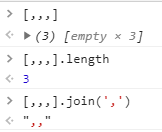# 36. class关键字

```var a = {class: "Animal", name: 'Fido'};
console.log(a.class); ```

# 37. 时间转换问题

```var a = new Date("epoch")
console.log(a); ```

new Date()构造函数传入的必须是一个时间字符串，即可以通过Date.parse()解析成功。所以本题输出Invalid Date。

# 38. 函数的形参个数

```var a = Function.length,
b = new Function().length
console.log(a === b); ```

Function 构造器本身也是个Function。他的length属性值为 1，所以a=1。该属性 Writable: false, Enumerable: false, Configurable: true。但是new Function()是一个对象，他没有形参，说以b=0，本题输出false。另外函数的实参的个数可以用argument.length获取。

# 39. 还是时间转换问题

```var a = Date(0);
var b = new Date(0);
var c = new Date();
console.log([a === b, b === c, a === c]); ```

# 40. Math.max()&Math.min()

```var min = Math.min(), max = Math.max()
console.log(min < max); ```

# 41. 正则表达式的“记忆”

```function captureOne(re, str) {
var match = re.exec(str);
return match && match;
}
var numRe  = /num=(\d+)/ig,
wordRe = /word=(\w+)/i,
a1 = captureOne(numRe,  "num=1"),
a2 = captureOne(wordRe, "word=1"),
a3 = captureOne(numRe,  "NUM=2"),
a4 = captureOne(wordRe,  "WORD=2");
console.log([a1 === a2, a3 === a4]);```

```var myRe = /ab*/g;
var str = 'abbcdefabh';
console.log(myRe.exec(str));
console.log(myRe.exec(str)); ```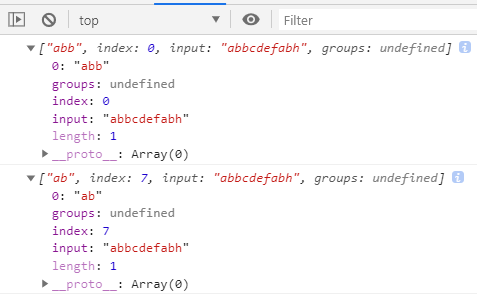# 42. Date中的month

```var a = new Date("2014-03-19"),
b = new Date(2014, 3, 19);
console.log([a.getDate() === b.getDate(), a.getMonth() === b.getMonth()]); ```

`new Date(year, monthIndex [, day [, hours [, minutes [, seconds [, milliseconds]]]]]); `

# 43. 正则表达式中的转义

```if ('http://giftwrapped.com/picture.jpg'.match('.gif')) {
console.log('a gif file');
} else {
console.log('not a gif file');
} ```

String.prototype.match 接受一个正则, 如果不是, 按照 new RegExp(obj) 转化. 所以 . 并不会转义，所以开头的‘http://gifwrapped......’中/gif就匹配了 /.gif/，所以输出“a gif file”

# 44. 变量提升

```function foo(a) {
var a;
return a;
}
function bar(a) {
var a = 'bye';
return a;
}
console.log([foo('hello'), bar('hello')]); ```

JavaScript语法本来就有很多不合理的地方，导致书写JavaScript很容易出错，本文中如有错误，欢迎各位看官提出来。